Processing ......FreeComputerBooks.com Links to Free Computer, Mathematics, Technical Books all over the World

R Programming for Data Science
🌠 Top Free C Programming Books - 100% Free or Open Source
• Title: R Programming for Data Science
• Author(s) Roger D. Peng
• Publisher: lulu.com; 5th Edition (April 20, 2016); eBook (Free Online Edtion, 2022-05-31)
• Hardcover/Paperback: 194 pages
• eBook: HTML
• Language: English
• ISBN-10/ASIN: 1365056821
• ISBN-13: 978-1365056826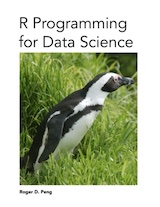Book Description

Data science has taken the world by storm. Every field of study and area of business has been affected as people increasingly realize the value of the incredible quantities of data being generated. But to extract value from those data, one needs to be trained in the proper data science skills.

The R programming language has become the de facto programming language for data science. Its flexibility, power, sophistication, and expressiveness have made it an invaluable tool for data scientists around the world.

This book is about the fundamentals of R programming. You will get started with the basics of the language, learn how to manipulate datasets, how to write functions, and how to debug and optimize code. With the fundamentals provided in this book, you will have a solid foundation on which to build your data science toolbox.

• N/A
Reviews, Rating, and Recommendations: Related Book Categories: Read and Download Links:Similar Books:
•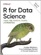R for Data Science: Visualize, Model, Transform, Tidy, Import

This book teaches you how to do data science with R: You'll learn how to get your data into R, get it into the most useful structure, transform it, visualize it and model it, how data science can help you work with the uncertainty and capture the opportunities.

•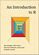An Introduction to R (Alex Douglas, et al.)

The main aim of this book is to help you climb the initial learning curve and provide you with the basic skills and experience (and confidence!) to enable you to further your experience in using R.

•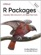R Packages: Organize, Test, Document, and Share Your Code

Turn your R code into packages that others can easily download and use. This practical book shows you how to bundle reusable R functions, sample data, and documentation together by applying author's package development philosophy.

•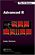Advanced R Programming (Hadley Wickham)

This book presents useful tools and techniques for attacking many types of R programming problems. It not only helps current R users become R programmers but also shows existing programmers what's special about R.

•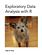Exploratory Data Analysis with R (Roger D. Peng)

This book covers the essential exploratory techniques for summarizing data with R. These techniques are typically applied before formal modeling commences and can help inform the development of more complex statistical models.

•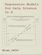Regression Models for Data Science in R (Brian Caffo)

The book gives a rigorous treatment of the elementary concepts of regression models from a practical perspective. The ideal reader for this book will be quantitatively literate and has a basic understanding of statistical concepts and R programming.

•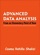Advanced Data Analysis using R (Cosma R. Shalizi)

This is a textbook on data analysis methods, intended for advance undergraduate students who have already taken classes in probability, mathematical statistics, and linear regression. All examples implemented using R.

•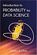Introduction to Probability for Data Science (Stanley Chan)

This book is an introductory textbook in undergraduate probability in the context of data science to emphasize the inseparability between data (computing) and probability (theory) in our time, with examples in both MATLAB and Python.

•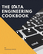Data Engineering Cookbook: The Plumbing of Data Science

This is a practical and comprehensive guide. You will learn the basics of data engineering. Then you will learn the technologies and frameworks required to build data pipelines to work with large datasets.

•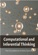Computational and Inferential: The Foundations of Data Science

Step by step, you'll learn how to leverage algorithmic thinking and the power of code, gain intuition about the power and limitations of current machine learning methods, and effectively apply them to real business problems.

•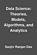Data Science: Theories, Models, Algorithms, and Analytics

It provides a bucket full of information regarding Data Science, covers a wide variety of sections by giving access to theories, data science algorithms, tools and analytics. You'll explore the right approach to best practices to guide you along the way.

•The Data Science Handbook: Advice and Insights

This book covers the essential exploratory techniques for summarizing data with R. These techniques are typically applied before formal modeling commences and can help inform the development of more complex statistical models.

•Python Data Science Handbook: Essential Tools (Jake VanderPlas)

Several resources exist for individual pieces of this data science stack, but only with the Python Data Science Handbook do you get them all - IPython, NumPy, Pandas, Matplotlib, Scikit-Learn, and other related tools.

Book Categories
 :All CategoriesTop Free BooksRecent BooksMiscellaneous BooksComputer EngineeringComputer LanguagesComputer ScienceData Science/DatabasesJava and Java EE (J2EE)Linux and UnixMathematicsMicrosoft and .NETMobile ComputingNetworking and CommunicationsSoftware EngineeringSpecial TopicsWeb Programming
Other Categories